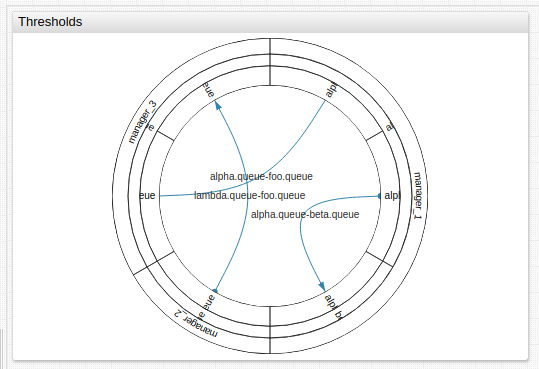# # Graph Widget

## # Overview

The Graph Widget displays the topology and relationships of servers, virtual machines, and managers alongside corresponding statuses.

``````[widget]
type = graph
timespan = 1 year
metric = uk-air.total_pax_this_period

[node]
id = HEATHROW
[node]
id = GLASGOW
[node]
id = MANCHESTER
``````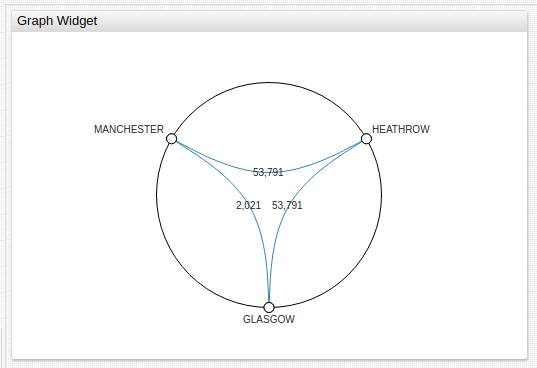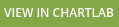## # Widget Settings

• The settings apply to the `[widget]` section.
• Common `[widget]` settings are inherited.
Name Description
`mode` Determines the overall look of the widget.
Possible values: `hierarchy`, `non-hierarchy`.
Default value: `non-hierarchy`.
Example: `mode = hierarchy`
`layout` Widget layout in `non-hierarchy` mode.
Possible values: `circle`, `rectangle`.
Default value: `circle`.
Example: `layout = rectangle`
`tension` Edge degree curvature.
Corresponds to the parameter `C` in the formula for the cardinal spline.
Possible values: `0` (straight line) to `1`.
Default value: `0.9`.
Example: `tension = 0.33`
`duration` The duration of a animation during graph geometry change, in milliseconds.
Default value: `1000`.
Example: `duration = 1500`
`arrows` Arrows on directed edges
Possible values: `false`, `true`.
Default value: `false`.
Example: `arrows = true`
`node-connect` Connect vertices on the perimeter
Possible values: `false`, `true`.
Default value: `true`.
Example: `node-connect = false`
`link-thresholds` Threshold for time series attached to the edges.
Supports both JavaScript and `percentile` functions.
Example: `link-thresholds = percentile(50), percentile(95)`
`link-colors` Color to indicate the threshold of time series.
Attached to the edges and separated by commas.
Example: `link-colors = red, yellow, green`
`link-widths` Width to indicate the thresholds of the time series tied to the edges and separated by commas or spaces.
Example: `link-widths = 5`
`node-thresholds` Threshold for time series tied to vertices.
Supports JavaScript, `percentile` functions, or can be set as an array.
Example: `node-thresholds = percentile(10),percentile(95)`
`node-colors` Color to indicate the threshold of time series that are bound to vertices and separated by commas.
Example: `node-colors = red, yellow, green`
`node-radiuses` Radii of the lighting for the display of the time series thresholds linked to the vertices and separated by commas or spaces.
Example: `node-radiuses = 10, 20, 30, 40`
`node-radius` Radius of the vertex in pixels.
Not valid in `hierarchy` mode.
Example: `node-radius = 10`
`padding` Offset from the edge of the container in pixels.
Example: `padding = 50`
`disconnected-node-display` Show vertices without edge.
Possible values: `false`, `true`.
Default value: `true`.
Example: `disconnected-node-display = false`
`node-labels` Display vertex labels.
Possible values: `false`, `true`.
Default value: `false`.
Example: `node-labels = false`
`link-labels` Display edge labels.
Possible values: `false`, `true`.
Default value: `false`.
Example: `link-labels = true`
`link-data` Series last value next to the `link-label`.
Example: `link-data = true`
`link-color-range` Color scheme for `link-threshold`.
Possible settings: `red`, `blue`, `black`.
Example: `link-color-range = red`
`link-label-zoom-threshold` Parameter value is a value of scale when labels appear based on edge threshold.
`value > 1`: Labels appear on zoom in.
Example: `link-label-zoom-threshold = 2`
`node-label-zoom-threshold` Parameter value is a value of scale when labels appear based on vertex threshold.
`value > 1`: Labels appear on zoom in.
Example: `node-label-zoom-threshold = 2`
`auto-padding` Add padding if labels overflow container.
Possible values: `false`, `true`.
Default value: `true`.
Example: `auto-padding = false`
`link-width-order` Sort links according to their width.
Possible values: `top`, `bottom`, `undefined`.
Default value: `undefined`.
Example: `link-width-order = top`

### # Controls

Name Description
`node-data` Show or hide time series last value information next to the corresponding image of the vertices.
Possible values: `false`, `true`.
Example: `data = true`
`depth` Set the depth of the displayed vertex hierarchy.
Specify a value from `1` to a maximum height of the vertex tree plus `1`, the last level corresponds to the edges.
Possible values: Number.
Default value: Equal to the height of the displayed vertex tree.
Example: `depth = 3`
`bundle` Hierarchical contraction of edges (Danny Holten algorithm).
Possible values: `false`, `true`.
Default value: `true`.
Example: `bundle = false`
`effects` Animation when changing the geometry of the graph.
Possible values: `false`, `true`.
Default value: `false`.
Example: `effects = true`
`node-collapse` Collapse sector peaks when clicked.
Possible values: `false`, `true`.
Default value: `true`.
Example: `node-collapse = true`
`link-animate` Animation of directed edges.
Possible values: `false`, `true`.
Default value: `false`.
Example: `link-animate = true`

## # Node Settings

• The settings apply to the `[node]` section.
Name Description
`id` Required Unique name of the vertex.
Example: `id = HEATHROW`
`parent` ID of parent vertex.
Example: `parent = HEATHROW`
• The settings apply to the `[link]` section.
Name Description
`id` Unique edge name.
Example: `id = HEATHROW - GLASGOW`
`nodes` Vertices connected by an edge.
ID of vertices, separated by a comma or hyphen for undirected edges, `-}` or `{-` for directed edges.
If vertex ID contains a hyphen, it must be shielded using `""` double quotes.
Example: `nodes = HEATHROW-MANCHESTER`
`series` `alias` of time series associated with the edge.
Example: `series = s1`
`tooltip` Link tooltip.
Example: `tooltip = Passenger Information`
`label` Label text.
Default value: `element-id`.
Example: `label = Airport Usage`

### # Series Settings

• The settings apply to the `[series]` section.
• Common `[series]` settings are inherited.
Name Description
`links` List of edges associated with this series is defined by an ID or edge ID of the corresponding vertices with a hyphen.
When edge ID contains a hyphen, it must be shielded with `""` double quotes.
Example: `links = HEATHROW - MANCHESTER`
`node-alert-style` Style of vertices when `alert-expression` is `true`.
Example: `node-alert-style = fill: red`
`link-alert-style` Style of edges when `alert-expression` is `true`.
Example: `link-alert-style = stroke: red`

### # Properties Settings

• The settings apply to the `[property]` section.
• In `[node]` or `[series]` section, define properties displayed on mouseover.
• If a value contains `=` or `\` escape them with a backslash: `\=` or `\\`, respectively.
• Use the `>` context setting displayed in the tooltip before the `[properties]` tooltip table.
• Within the `>` context setting, create a table using standard HTML parameters: `>` table class, `tbody`, `tr`, `td`.
``````[properties]
name = QM-2
code = 003
``````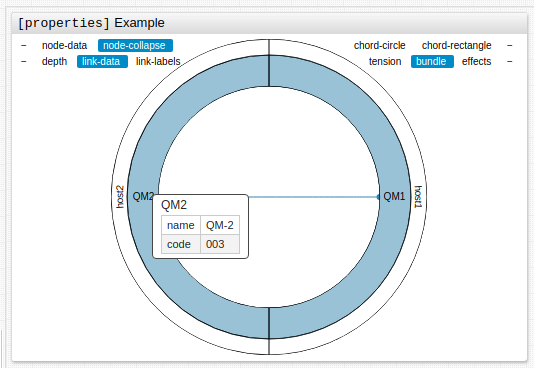## # Examples

### # Structure

The structure of the graph is defined by the description of its vertices and edges. Use `[node]` settings to describe each vertex, and define the hierarchy of vertices with the `parent` setting, which sets the predecessor. Use `[link]` settings to describe the edges. Adjacent edges, with vertices, are determined by `[node]` settings.

Link series to edges or vertices by indicating the `alias` in the series parameter of `[link]` or `[node]` settings. Alternatively, list the loaded edges or vertices in the links or nodes of the `[series]` settings.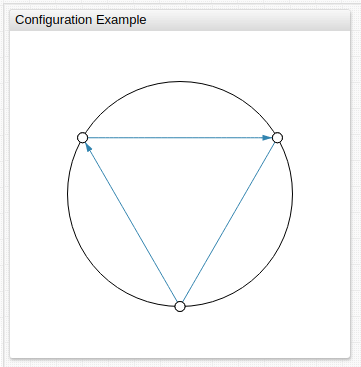In the event of an `alert-expression` response on the time series of the vertex or edge to which the time series is tied. `alert-style` setting value can be either string or script. If `alert-style` is script, the variable alert is available, and it is equal to the value that the alert returns. The following styles are applied (in a specified order) in the parameters below:

### # Example Syntax

``````/* Modify stroke width of series line on alert */

/* modify color of node on alert */
``````

### # Configuration Example 2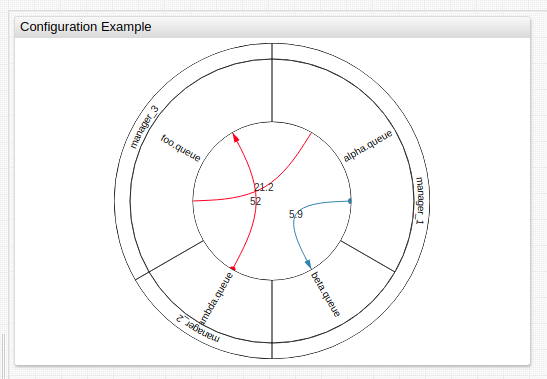## # Thresholds

Set thresholds for time series which are tied to vertices or edges using `link-thresholds` or `node-thresholds` parameters in `[widget]` settings.

Color and width parameters are applied to the edge and vertices based on `link-widths` and `link-colors`, additionally `node-radiuses` and `node-colors` in `[widget]` settings.

`alert-expression` styles are superimposed over threshold styles.